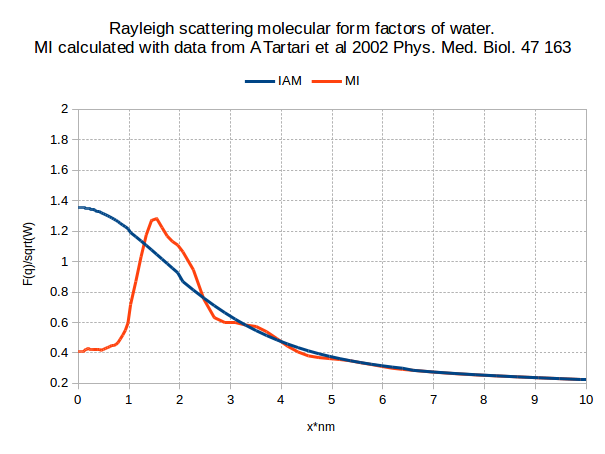# Rayleigh scattering form factors from PENELOPE appear lower than literature

Dear all,

I instantiate a water material
`G4NistManager* nist = G4NistManager::Instance();`
`G4Material* mymat = nist->FindOrBuildMaterial("G4_WATER")`

and then, using `G4PenelopeRayleighModel::DumpFormFactorTable(mymat)`, I retrieve the Rayleigh scattering form factors which are calculated according to the independent atom model(IAM).

I then edit the form factors to add molecular interference (MI) effects and retrieve them back again to plot the MI form factors in comparison with the IAM form factors.

Common practice has it that the plots be normalised(divided) by sqrt(W), where W is the molecular weight of water but if I do that using 18.01528 g/mol then the results(first figure) are half of what they are reported in the literature(second figure)***SEE EDIT BELOW Geant4 implementation of inter-atomic interference effect in small-angle coherent X-ray scattering for materials of medical interest.I notice units of “mol” missing from the y axis(W has units of g/mol and F has no units) in the literature so I am wondering why they disappear and if this is the reason why both Geant4 IAM and my MI form factors are half of those in the literature.

***EDIT
Results are not half. It is that if I use 9 g/mol instead of 18 g/mol that makes the results be equal.

Many thanks,
Thomas

The reason behind this discrepancy is the way `G4PenelopeRayleighModel.cc` calculates the form factor.

The Independent Atom Model states that for a material with molecular weight W, comprised of `N` elements with atomic weight `A_i`, mass fraction `w_i` and atomic form factor `f_i`, the molecular form factor is
`F(x)^2 = W * Sum[ (w_i/A_i) * f_i(x)^2 ]`
and so
`F(x)^2/W = Sum[ (w_i/A_i) * f_i(x)^2 ] Eq(1)`

On the other hand, `G4PenelopeRayleighModel.cc` (probably all models in general) calculates the form factor according to the IAM model a little differently:
`F'(x)^2 = Sum[ (w_i/A_i) * f_i(x)^2 ] / Max(w_i/A_i)`
and so
`F'(x)^2 * Max(w_i/A_i) = Sum[ (w_i/A_i) * f_i(x)^2 ]`
and from Eq(1) we get
`F'(x)^2 * Max(w_i/A_i) = F(x)^2/W`

So, when I Get the form factor table with `G4PenelopeRayleighModel::DumpFormFactorTable`, I get F’ so I have to multiply by `SQRT(Max(w_i/A_i))` to get `F/SQRT(W)`.